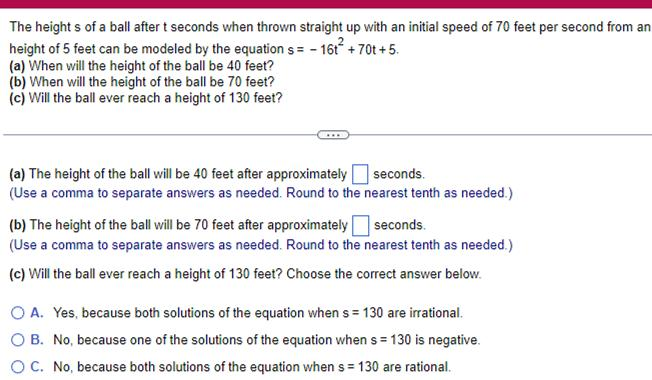Question:

# The heights of a ball after t seconds when thrown straight

Last updated: 9/20/2023The heights of a ball after t seconds when thrown straight up with an initial speed of 70 feet per second from an height of 5 feet can be modeled by the equation s 16t 70t 5 a When will the height of the ball be 40 feet b When will the height of the ball be 70 feet c Will the ball ever reach a height of 130 feet a The height of the ball will be 40 feet after approximately seconds Use a comma to separate answers as needed Round to the nearest tenth as needed b The height of the ball will be 70 feet after approximately seconds Use a comma to separate answers as needed Round to the nearest tenth as needed c Will the ball ever reach a height of 130 feet Choose the correct answer below O A Yes because both solutions of the equation when s 130 are irrational O B No because one of the solutions of the equation when s 130 is negative O C No because both solutions of the equation when s 130 are rational